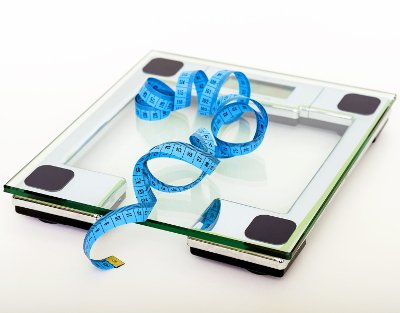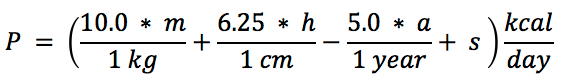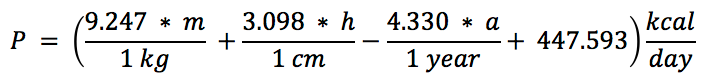# Online BMR (Basal Metabolic Rate) Calculator

Share:

Enter your gender, age, height and weight and then click the "Calculate BMR" button.

*
*
*
ft
Feet
in
Inches
cm
Centimeters
*
lb
Pounds
kg
Kilograms

Similar Fitness Calculators

## About BMR (Basal Metabolic Rate) CalculatorThis online Basal Metabolic Rate (BMR) calculator takes your gender, age, weight and height and calculates the BMR in kcal/day. Basal Metabolic Rate (BMR) is used to identify the calorie required by the body to do its basic functions like breathing, cell growth, blood circulation, etc. at rest.

### Using the Basal Metabolic Rate (BMR) calculator

3. Enter your Height either in centimeters or in feet.
4. Enter your Weight either in kilogram (kg) or in pounds (lb.).
5. Chose the formula for the calculation either Harris-Benedict Equation or Mifflin St Jeor Equation
6. Then, click the Calculate BMR button. The Basal Metabolic Rate will be calculated and the result will be displayed in the box below the button.

## BMR (Basal Metabolic Rate)

Basal Metabolic Rate (BMR) is the measurement of the energy consumed by the human body in rest to perform its basic functions like breathing, blood circulation, etc...

Basal Metabolic Rate (BMR) has several calculation formulas. The popular ones are The Mifflin St Jeor Equation and the The Revised Harris-Benedict Equation.

### The Mifflin St Jeor Equation• P = Total energy consumed at rest in kcal per day.
• s = A constant. Substitute +5 for men and -161 for women.
• m = Mass in kilogram (kg).
• h = Height in centimeters (cm).
• a = Age in years.

### The Revised Harris-Benedict Equation

The Revised Harris-Benedict Equation has 2 formulas, one for women and another for men.

#### Formula For Men• P = Total energy consumed at rest in kcal per day.
• m = Mass in kilogram (kg).
• h = Height in centimeters (cm).
• a = Age in years.

#### Formula For Women• P = Total energy consumed at rest in kcal per day.
• m = Mass in kilogram (kg).
• h = Height in centimeters (cm).
• a = Age in years.